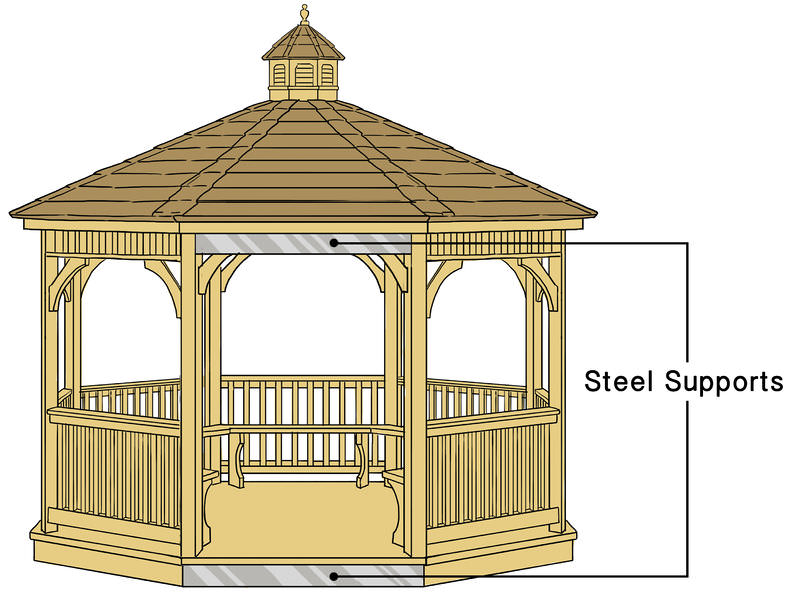# 1.6.4: When to use the Distributive Property

•• Contributed by CK12
• CK12

## When to Use the Distributive Property

David and Denise are having an argument. David says that you can't use the Distributive Property to simplify the expression (4x+5)/8, while Denise says that you can. Who do you think is right?

### Identifying Expressions Involving the Distributive Property

The Distributive Property often appears in expressions, and many times it does not involve parentheses as grouping symbols. Recall that the fraction bar acts as a grouping symbol.

#### Let's simplify the following expressions with fractions:

1. (9−6y)/3

The denominator needs to be distributed to each part of the expression in the numerator.

We can rewrite the expression so that we can see how the Distributive Property should be used:

(9−6y)/3=1/3(9−6y)=1/3(9)−1/3(6y)=(9/3)−(6y/3)=3−2y.

1. (2x+4)/8

Think of the denominator as 1/8: (2x+4)/8=1/8(2x+4).

Now apply the Distributive Property: 1/8(2x)+1/8(4)=2x/8+4/8.

Simplified: x/4+1/2.

#### Real-World Problems and the Distributive Property

The Distributive Property is one of the most common mathematical properties seen in everyday life. It crops up in business and in geometry. Anytime we have two or more groups of objects, the Distributive Property can help us solve for an unknown.

#### Let's use the Distributive Property to solve the following problem:

An octagonal gazebo is to be built as shown below. Building code requires five-foot-long steel supports to be added along the base and four-foot-long steel supports to be added to the roof-line of the gazebo. What length of steel will be required to complete the project?Figure $$\PageIndex{1}$$

Each side will require two lengths, one of five and one of four feet respectively. There are eight sides, so here is our equation.

Steel required =8(4+5) feet.

We can use the Distributive Property to find the total amount of steel.

Steel required =8×4+8×5=32+40 feet.

A total of 72 feet of steel is needed for this project.

### Examples

Example $$\PageIndex{1}$$

Earlier, you were told that David says that you can't use the Distributive Property to simplify the expression (4x+5)/8, while Denise says that you can. Who is correct?

Solution

Denise is correct, you can use the Distributive Property to simplify the expression. You can distribute the denominator.

(4x+5)/8=1/8(4x+5)=1/8(4x)+1/8(5)=x/2+5/8

The simplified form of the expression is x/2+5/8.

Example $$\PageIndex{1}$$

Simplify (10x+8y−1)/2.

Solution

First we rewrite the expression so we can see how to distribute the denominator:

(10x+8y−1)/2=1/2(10x+8y−1)=1/2(10x)+1/2(8y)−1/2(1)=5x+4y−12

### Review

Use the Distributive Property to simplify the following expressions.

1. (2−j)(−6)
2. (r+3)(−5)
3. 6+(x−5)+7

Use the Distributive Property to simplify the following fractions.

1. (8x+12)/4
2. (9x+12)/3
3. (11x+12)/2
4. (3y+2)/6
5. (−6z−2)/3
6. (7−6p)/3

In 10 – 17, write an expression for each phrase.

1. 2/3 times the quantity of n plus 16
2. Twice the quantity of m minus 3
3. −4x times the quantity of x plus 2
4. A bookshelf has five shelves, and each shelf contains seven poetry books and eleven novels. How many of each type of book does the bookcase contain?
5. Use the Distributive Property to show how to simplify 6(19.99) in your head.
6. A student rewrote 4(9x+10) as 36x+10. Explain the student’s error.
8. Amar is making giant holiday cookies for his friends at school. He makes each cookie with 6 oz of cookie dough and decorates each one with macadamia nuts. If Amar has 5 lbs of cookie dough (1 lb=16 oz)and 60 macadamia nuts, calculate the following.
1. How many (full) cookies can he make?
2. How many macadamia nuts can he put on each cookie if each is supposed to be identical?Figure $$\PageIndex{1}$$